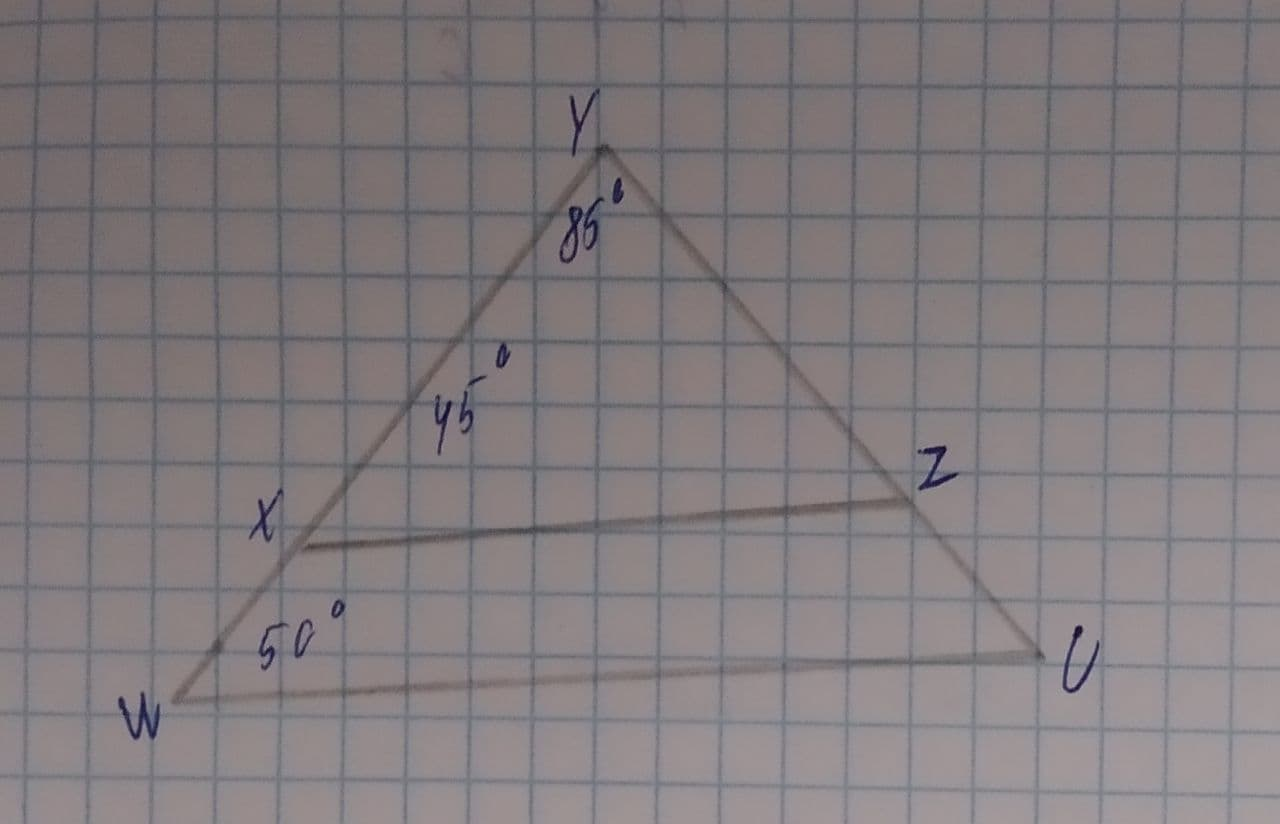# Write the triangle similarity statement and the reason for why the triangles \triangle XYZ\sim \triangle ? By: ? are similar.Ernstfalld 2021-08-11 Answered
Write the triangle similarity statement and the reason for why the triangles are similar.$$\displaystyle\triangle{X}{Y}{Z}\sim\triangle?{B}{y}:?$$

• Questions are typically answered in as fast as 30 minutes

### Plainmath recommends

• Get a detailed answer even on the hardest topics.
• Ask an expert for a step-by-step guidance to learn to do it yourself.lamusesamuset
Step 1
Taking $$\displaystyle\triangle{X}{Y}{Z}$$
$$\displaystyle\angle{X}={45}^{{\circ}},\angle{Y}={85}^{{\circ}}$$
Now,
Since sum of interior angle of triangle is $$\displaystyle{180}^{{\circ}}$$
$$\displaystyle\Rightarrow\angle{X}+\angle{Y}+\angle{Z}={180}^{{\circ}}$$
$$\displaystyle{45}^{{\circ}}+{85}^{{\circ}}+\angle{Z}={180}^{{\circ}}$$
$$\displaystyle\angle{Z}={180}^{{\circ}}-{130}^{{\circ}}$$
$$\displaystyle\angle{Z}={50}^{{\circ}}$$
Step 2
Taking $$\displaystyle\triangle{W}{Y}{U}$$
$$\displaystyle\angle{W}={50}^{{\circ}},\angle{Y}={85}^{{\circ}}$$
Now,
Since sum of interior angle of triangle is $$\displaystyle{180}^{{\circ}}$$
$$\displaystyle\Rightarrow\angle{W}+\angle{Y}+\angle{U}={180}^{{\circ}}$$
$$\displaystyle{50}^{{\circ}}+{85}^{{\circ}}+\angle{U}={180}^{{\circ}}$$
$$\displaystyle\angle{U}={180}^{{\circ}}-{135}^{{\circ}}$$
$$\displaystyle\angle{U}={45}^{{\circ}}$$
Step 3
In $$\displaystyle\triangle{X}{Y}{Z}\ {\quad\text{and}\quad}\ \triangle{U}{Y}{W}$$
$$\displaystyle\angle{X}=\angle{U}={50}^{{\circ}}$$
$$\displaystyle\angle{Y}=\angle{Y}={85}^{{\circ}}$$
$$\displaystyle\angle{Z}=\angle{W}={45}^{{\circ}}$$
Therefore
$$\displaystyle\triangle{X}{Y}{Z}\sim\triangle{U}{Y}{W}$$ By: AA similarity